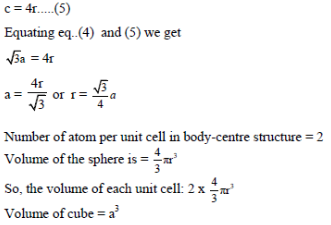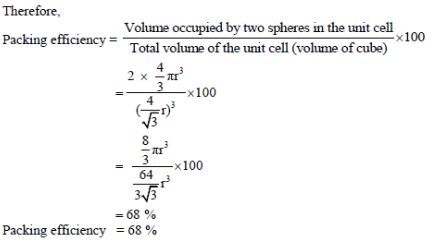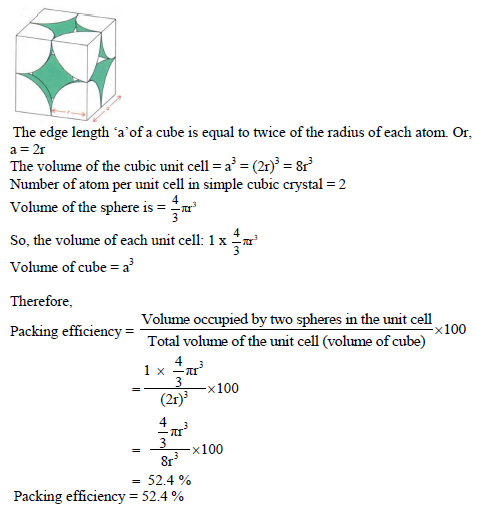Now consider the unit cell of body-centred cubic in which sphere ABCDEFGH are occupied at corner. And sphere c is placed at body-centre of unit cell as shown below:The length of the body diagonal c is equal to 4r, where r is the radius of the sphere (atom). Therefore,Packing efficiency in the simple cubic lattice:

In the unit cell of simple cubic lattice, the atoms are occupied only at corner of unit cells.